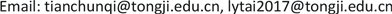1同济大学计算机科学与技术系，上海

2华东师范大学数据科学学院，上海1. 引言

2. 相关工作

Zhao X，Kc K   等人基于源代码分析日志的文法结构，形成正则表达式。文献  通过分析原始日志并结构化来发现应用程序日志中的模式，它从一组表示系统正常运行的日志中发现一组GROK模式，然后使用这些GROK模式来解析日志。

3. 异常检测模型3.1. 微服务系统日志数据预处理

3.1.1. 微服务系统日志解析

3.1.2. 微服务系统日志划分

3.2. 建立模型

3.2.1. 提取微服务系统日志键序列

3.2.2. LSTM异常检测模型

Pr ( d t = k | y t ) = e y t k ∑ i = 1 k e y t k (1)

C = − ∑ i = 1 K w k × [ d t i log ( y t i ) + ( 1 − d t i ) log ( 1 − y t i ) ] (2)

4. 实验及分析4.1. 实验数据准备

EFK是由一套开源软件组成的日志解决方案，它包括三个组件：Elasticsearch, Fluentd, Kibana。Elasticsearch是一个分布式的日志存储和日志搜索引擎，通过Restful方式进行交互，Fluentd负责收集日志发送给 Elasticsearch, Kibana可以将Elasticsearch中的数据通过友好的界面展示出来。我们通过EFK来收集在线实训平台系统产生的海量日志，当系统管理员发现问题时，会记录该异常数据。

4.2. 准确率

Confusion matri

Positive (1)True Positive (TP)False Negative (FN)
Negative (0)False Positive (FP)True Negative (TN)

p r e c i s i o n = T P T P + F P (3)

r e c a l l = T P T P + F N (4)

F 1 = 2 × p r e c i s i o n × r e c a l l p r e c i s i o n + r e c a l l (5)

4.3. 日志键序列的长度

4.4. 训练数据集的大小

5. 结论